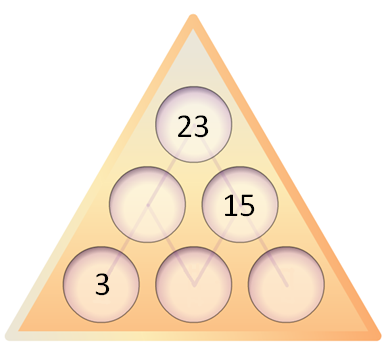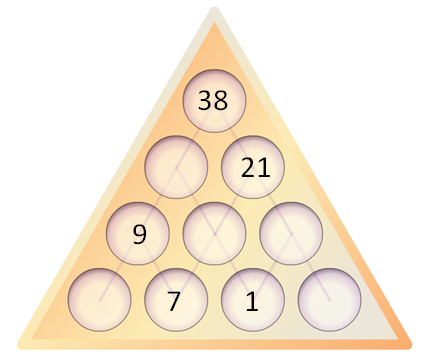# Number PyramidsIn a Number Pyramid, the numbers on the lower levels determine the numbers above them.

Choose three single-digit numbers and enter them in the bottom row of the interactive Number Pyramids.

Try entering some different numbers in the bottom row.
Can you work out how the numbers in the upper layers are generated?

Here are some questions to consider:

If I tell you the numbers on the bottom layer, can you work out the top number without working out the middle layer?

If you change the order of the numbers on the bottom layer, will the top number change?

Given any three numbers for the bottom, how can you work out the largest possible number that could go at the top?

If I give you a target for the top number, can you quickly find three possible numbers for the bottom?

Can you adapt what you learned about 3-layer pyramids to larger pyramids?

Here is an interactive Number Pyramid with four layers so you can test out your ideas.

You can create a Number Pyramid puzzle by erasing numbers from a completed Number Pyramid and challenging someone to find the missing numbers.
Here's a couple for you to try:What is the minimum number of cells that need to be filled, in order for a puzzle to have a unique solution?
Does it matter which cells?